# What is a Coordinate Plane? - Definition, Quadrants & Example

An error occurred trying to load this video.

Try refreshing the page, or contact customer support.

Coming up next: Circles: Area and Circumference

### You're on a roll. Keep up the good work!

Replay
Your next lesson will play in 10 seconds
• 0:02 Confused Students
• 1:33 Coordinate Plane
• 2:55 Points in Action
• 4:06 Homework
• 5:14 Lesson Summary

Want to watch this again later?

Timeline
Autoplay
Autoplay
Speed

#### Recommended Lessons and Courses for You

Lesson Transcript
Instructor: Joseph Vigil
In this lesson, you'll learn what a coordinate plane is and some coordinate plane terminology. You'll also see a few examples of coordinate planes in action. Then, you can test your new knowledge with a brief quiz.

## Confused Students

Mrs. Velasquez gave her class a number line to plot different values.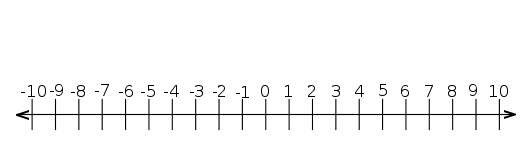She explained to them that the arrowheads mean that the number line goes on forever. But they're just going to work with the numbers between -10 and 10 today. She told the class that a square is 6 feet long and asked them to plot that measurement on the number line.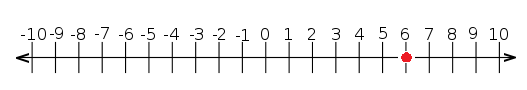Then, she told them that an apple tree dropped 2 apples. She asked them to plot this situation on the number line. The students added that point to their number lines.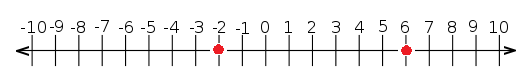Now, Mrs. Velasquez had a trick up her sleeve. She told her students that a triangle has a length of 6 inches and a height of 2 inches. Some students plotted only the length.Some plotted only the height.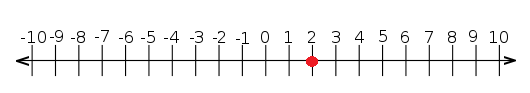Some tried plotting both values on the same number line.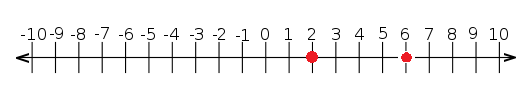Mrs. Velasquez told the class that didn't quite work because length and height are different values. The class was stumped, so she asked them, 'What if I added another number line so you can plot both numbers?' She then showed them a picture of a coordinated plane.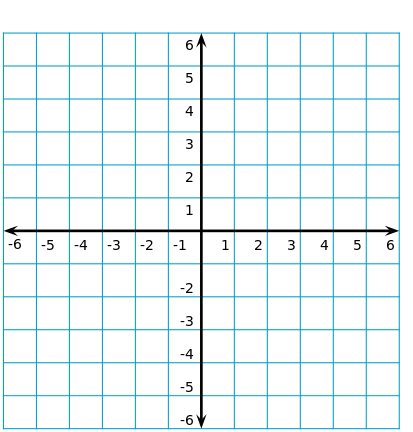A collective, 'Ah!' arose from the students. They now had two number lines that would allow them to plot two different values. Mrs. Velasquez said they would use the number line going left to right to represent length and the one going up and down to represent height. She demonstrated by moving six units to the right on the horizontal number line, then she moved two units up on the vertical number line. She plotted the point where she ended up. She has now plotted the mystery point.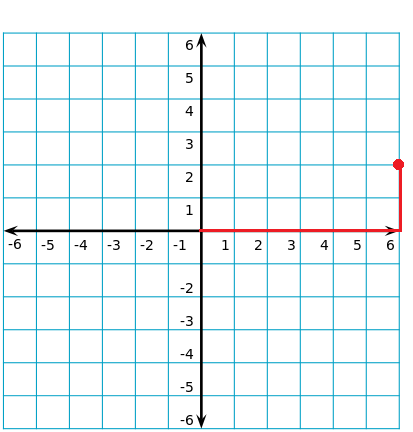## Coordinate Plane

What Mrs. Velasquez did was create a coordinate plane, which is an intersection of two number lines. Just like a regular number line, the ones on a coordinate plane could stretch on infinitely. So we can choose a stopping plot on the number lines convenient for a specific situation.

For example, if the triangle's height were 100 inches, Mrs. Velasquez could have made each number line go up to 100. But since she was only going up to 6, number lines ending at 6 were sufficient. The length of a coordinate plane's number lines is known as its scale.

For people to use coordinate planes, they need to have a common language. By convention, we normally call the horizontal number line the x-axis, and we normally call the vertical number line the y-axis.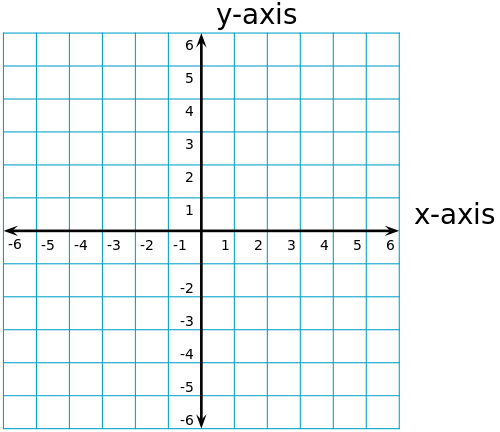Notice that the axes cut the coordinate plane into four equal sections, or quadrants.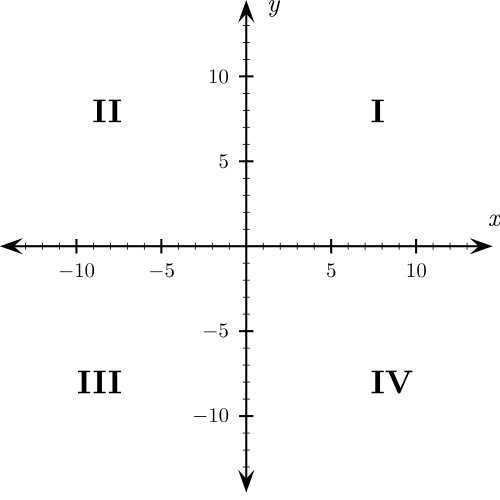So the point that Mrs. Velasquez plotted above is in quadrant I. The number of units you move along the axes are the point's coordinates. Since you moved 6 positive units along the x-axis, the point's x-coordinate is 6. Since you moved 2 positive units along the y-axis, the point's y-coordinate is 2.

To name a point, we use its x-coordinate followed by its y-coordinate in parentheses. For example, this point would be named (6, 2) since 6 is its x-coordinate and 2 is its y-coordinate.

## Points in Action

Mrs. Velasquez showed the class this point: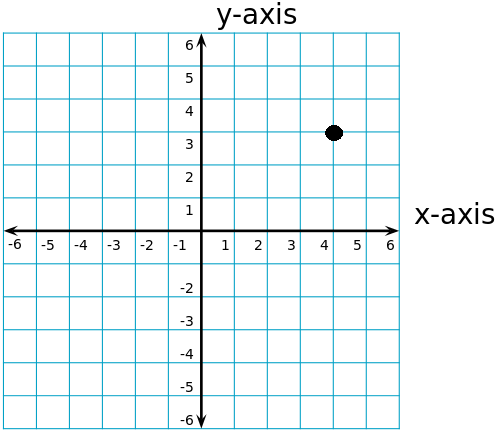She asked them to name it. After a few seconds counting along the number lines, one student called out '(3, 4).'

To unlock this lesson you must be a Study.com Member.

### Register to view this lesson

Are you a student or a teacher?

### Unlock Your Education

#### See for yourself why 30 million people use Study.com

##### Become a Study.com member and start learning now.
Back
What teachers are saying about Study.com

### Earning College Credit

Did you know… We have over 160 college courses that prepare you to earn credit by exam that is accepted by over 1,500 colleges and universities. You can test out of the first two years of college and save thousands off your degree. Anyone can earn credit-by-exam regardless of age or education level.